How PROC MCMC Works

Subsections:

PROC MCMC is a simulation-based procedure that applies a variety of sampling algorithms to the program at hand. The default sampling methods include conjugate sampling (from full conditional), direct sampling from the marginal distribution, inverse cumulative distribution function, random walk Metropolis with normal proposal, and discretized random walk Metropolis with normal proposal. You can request alternate sampling algorithms, such as random walk Metropolis with t distribution proposal, discretized random walk Metropolis with symmetric geometric proposal, and the slice sampling algorithm.

PROC MCMC applies the more efficient sampling algorithms first, whenever possible. When a parameter does not appear in the conditional distributions of other random variables in the program, PROC MCMC generates samples directly from its prior distribution (which is also its marginal distribution). This usually occurs in data-independent Monte Carlo simulation programs (see Simulating Samples From a Known Density for an example) or missing data problems, where the missing response variables are generated directly from the conditional sampling distribution (or the conditional likelihood). When conjugacy is detected, PROC MCMC uses random number generators to draw values from the full conditional distribution. (For information about detecting conjugacy, see the section Conjugate Sampling.) In other situations, PROC MCMC resorts to the random walk Metropolis with normal proposal to generate posterior samples for continuous parameters and a discretized version for discrete parameters. See the section Metropolis and Metropolis-Hastings Algorithms in Chapter 7: Introduction to Bayesian Analysis Procedures, for details about the Metropolis algorithm. For the actual implementation details of the Metropolis algorithm in PROC MCMC, such as tuning of the covariance matrices, see the section Tuning the Proposal Distribution.

A key component of the Metropolis algorithm is the calculation of the objective function. In most cases, the objective function that PROC MCMC uses in a Metropolis step is the logarithm of the joint posterior distribution, which is calculated with the inclusion of all data and parameters. The rest of this section describes how PROC MCMC calculates the objective function for parameters that use the Metropolis algorithm.

Model Parameters

To calculate the log of the posterior density, PROC MCMC assumes that all observations in the data set are independent,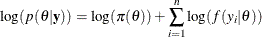whereis a parameter or a vector of parameters that are defined in the PARMS statements (referred to as the model parameters). The term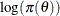is the sum of the log of the prior densities specified in the PRIOR and HYPERPRIOR statements. The term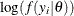is the log likelihood specified in the MODEL statement. The MODEL statement specifies the log likelihood for a single observation in the data set.

If you want to model dependent data—that is,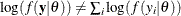—you can use the JOINTMODEL option in the PROC MCMC statement. See the section Modeling Joint Likelihood for more details.

The statements in PROC MCMC are similar to DATA step statements; PROC MCMC evaluates every statement in order for each observation. At the beginning of the data set, the log likelihood is set to be 0. As PROC MCMC steps through the data set, it cumulatively adds the log likelihood for each observation. Statements between the BEGINNODATA and ENDNODATA statements are evaluated only at the first and the last observations. At the last observation, the log of the prior and hyperprior distributions is added to the sum of the log likelihood to obtain the log of the posterior distribution.

Calculation of the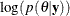objective function involves a complete pass through the data set, making it potentially computationally expensive. If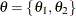is multidimensional, you can choose to update a portion of the parameters at each iteration step by declaring them in separate PARMS statements (see the section Blocking of Parameters for more information). PROC MCMC updates each block of parameters while holding others constant. The objective functions that are used in each update are the same as the log of the joint posterior density: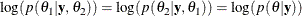In other words, PROC MCMC does not derive the conditional distribution explicitly for each block of parameters, and it uses the full joint distribution in the Metropolis step for every block update.

Random-Effects Models

For programs that require RANDOM statements, PROC MCMC includes the sum of the density evaluation of the random-effects parameters in the calculation of the objective function for,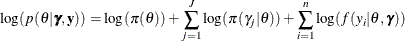where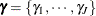are random-effects parameters and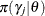is the prior distribution of the random-effects parameters. The likelihood function can be conditional on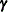, but the prior distributions of, which must be independent of, cannot.

The objective function used in the Metropolis step for the random-effects parameter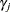contains only the portion of the data that belong to the jth cluster: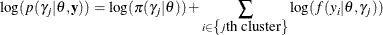The calculation does not include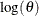, the prior density piece, because that is a known constant. Evaluation of this objective function involves only a portion of the data set, making it more computationally efficient. In fact, updating every random-effects parameters in a single RANDOM statement involves only one pass through the data set.

You can have multiple RANDOM statements in a program, which adds more pieces to the posterior calculation, such as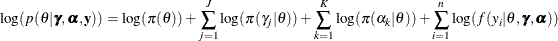where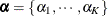is another random effect. The random effectsand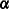can form their own hierarchy (as in a nested model), or they can enter the program in a non-nested fashion. The objective functions forand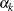are calculated using only observations that belong to their respective clusters.

Models with Missing Values

Missing values in the response variables of the MODEL statement are treated as random variables, and they add another layer in the conditional updates in the simulation. Suppose that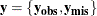The response variable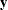consists of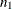observed values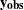and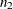missing values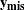. The log of the posterior distribution is thus formed by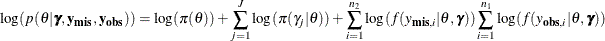where the expression is evaluated at the drawnandvalues.

The conditional distribution of the random-effects parameteriswhere theare either the observed or the imputed values of the response variable.

The missing values are usually sampled directly from the sampling distribution and do not require the Metropolis sampler. When a response variable takes on a GENERAL function, the objective function is simply the likelihood function: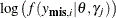.